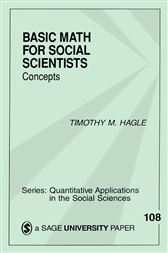Basic Math for Social Scientists

Series: Quantitative Applications in the Social Sciences (No. 108)US\$18.00
ISBNs
• 9780803958753
• 9781506317694
A review of the basic mathematical concepts that underlie most quantitative analysis in the social sciences is presented in this volume. The author begins with an algebra review featuring sets and combinations and then discusses limits and continuity. Calculus is presented next, with an introduction to differential calculus, multivariate functions, partial derivatives, and integral calculus. Finally the book deals with matrix algebra. Packed with helpful definitions, equations, examples and alternative notations, the book also includes a useful appendix of common mathematical symbols and Greek letters.

ISBNs
• 9780803958753
• 9781506317694
ISBNs
• 9780803958753
• 9781506317694